﻿ PEMFC分数阶子空间建模

# PEMFC分数阶子空间建模Research on Fractional Order Subspace Modeling

Abstract: Proton Exchange Membrane Fuel Cell (PEMFC) is a complex multi-variable, strongly coupled and non-linear system. Accurate modeling method is the basis of its research, and advanced control strategy is the key to improving the power generation performance. In recent years, many studies have shown that the dynamic processes of PEMFC power generation, such as gas diffusion, heat conduction and electrochemical reaction, have fractional order characteristics. In this paper, a fractional order state space model of PEMFC is established by combining fractional order theory with subspace identification method (SIM).

1. 引言

PEMFC是一个包括流动、传质、传热和电化学反应等多种物理化学现象的复杂机体，若要对其进行深入研究，建模是一种直观且快速的手段。根据建模方法划分，PEMFC模型可分为机理模型和经验模型。机理模型主要是针对电堆内部的气体、质子、水以及热量分布等进行建模，采用能量守恒方程、传质传热方程和电化学反应等方程来描述相应参数对PEMFC的输出特性的影响。Amphlett  提出了PEMFC的电化学模型，描述了阴极/阳极气体分压、温度和电流密度对输出电压的影响。朱柳  根据能量守衡定律，考虑了电堆自身的热辐射及欧姆极化的影响，构建了PEMFC的热管理模型。Zhang  研究了PEMFC电堆内部水的气、液两相分布问题，根据质量守恒定律，建立了PEMFC的水管理模型。此外，还有不少学者还考虑了反应物浓度、电堆温度、水、电流密度等因素在二维或者三维空间分布不均对PEMFC的影响，建立了更加精确和全面的PEMFC模型，但相应的模型也更加复杂      。机理建模需对PEMFC系统有大量的先验知识，理解相对复杂，但可以反映电池内部参数变化对输出特性的影响。机理模型建模过程复杂、模型参数众多且获取难度大，适合对电池性能进行分析，一般不适用于控制。

2. PEMFC概述

EMFC单电池结构如图1所示，主要由双极板、密封圈和膜电极组件(Membrane Electrodes Assembly，MEA)构成，其中MEA为PEMFC的核心部分，包括质子交换膜、阴/阳极催化层和气体扩散层。

PEMFC的工作原理实际上是水电解的逆过程，如图2所示。氢气经过气体流场注入阳极室，然后通过气体扩散层到达阳极催化层，在阳极催化剂的作用下，氢气分解为质子和电子，质子穿过交换膜到达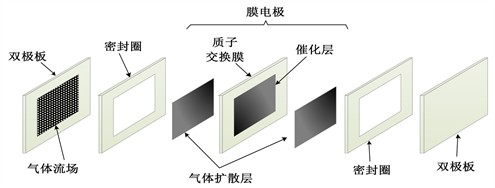Figure 1. Structural schematic diagram of PEMFC single cellFigure 2. Working principle diagram of PEMFC

$E=N\cdot \left({E}_{nernst}-{V}_{act}-{V}_{ohm}-V{}_{con}\right)$ (4)

${E}_{nernst}$ 可以由下式表示：

${E}_{nernst}=\frac{\Delta G}{2F}+\frac{\Delta S}{2F}\left(T-{T}_{ref}\right)+\frac{RT}{2F}\left(\mathrm{ln}{P}_{{H}_{2}}+\frac{1}{2}\mathrm{ln}{P}_{{O}_{2}}\right)$ (5)

${V}_{act}$ 表示电池电化学反应克服活化对外做功时带来的损耗，可以下式表示：

${V}_{act}={\eta }_{1}+{\eta }_{2}T+{\eta }_{3}T\mathrm{ln}{C}_{{O}_{2}}+{\eta }_{4}T\mathrm{ln}i$ (6)

${C}_{{O}_{2}}=\frac{{P}_{{O}_{2}}}{5.08×{10}^{6}×\mathrm{exp}\left(-498/T\right)}$ (7)

${V}_{ohm}$ 主要是由单电池等效内阻 ${R}_{c}$ 引起，满足欧姆定律，可表示为：

${V}_{ohm}=i\cdot {R}_{c}$ (8)

${R}_{c}$ 是与质子交换膜电阻率、电池材料、结构、工作温度以及湿度等因素相关。

${V}_{con}$ 是在电化学反应中，由于质量传输导致反应物浓度(氢气、氧气、水以及离子等)发生变化而产生的，其表达式如下：

${V}_{con}=-B×\mathrm{ln}\left(1-\frac{J}{{J}_{\mathrm{max}}}\right)$ (9)

3. PEMFC分数阶子空间建模

${}_{a}\mathcal{D}{}_{t}^{\alpha }=\left\{\begin{array}{l}\frac{{\text{d}}^{\alpha }}{\text{d}t},R\left(\alpha \right)>0\\ 1,R\left(\alpha \right)=0\\ {\int }_{a}^{t}{\left(\text{d}\tau \right)}^{-\alpha },R\left(\alpha \right)<0\end{array}$ (10)

${\mathcal{D}}^{{\alpha }_{n}}y\left(t\right)+{a}_{n-1}{\mathcal{D}}^{{\alpha }_{n-1}}y\left(t\right)+\cdots +{a}_{0}{\mathcal{D}}^{{\alpha }_{0}}y\left(t\right)={b}_{m}{\mathcal{D}}^{{\beta }_{m}}u\left(t\right)+{b}_{m-1}{\mathcal{D}}^{{\beta }_{m-1}}u\left(t\right)+\cdots {b}_{0}{\mathcal{D}}^{{\beta }_{0}}u\left(t\right)$ (11)

$\mathcal{L}\left[{\mathcal{D}}^{\alpha }f\left(t\right)\right]={s}^{\alpha }F\left(s\right)$ (12)

$G\left(s\right)=\frac{Y\left(s\right)}{U\left(s\right)}=\frac{{b}_{m}{s}^{{\beta }_{m}}+{b}_{m-1}{s}^{{\beta }_{m-1}}+\cdots +{b}_{0}}{{s}^{{\alpha }_{n}}+{a}_{n-1}{s}^{{\alpha }_{n-1}}+\cdots +{a}_{0}}$ (13)

$\left\{\begin{array}{l}{\mathcal{D}}^{\alpha }x\left(t\right)=Ax\left(t\right)+Bu\left(t\right)\hfill \\ y\left(t\right)=Cx\left(t\right)+Du\left(t\right)\hfill \end{array}$ (14)

$\left\{\begin{array}{l}{\mathcal{D}}^{\alpha }x\left(t\right)=Ax\left(t\right)+Bu\left(t\right)+w\left(t\right)\\ y\left(t\right)=Cx\left(t\right)+Du\left(t\right)+v\left(t\right)\end{array}$ (15)

$\begin{array}{c}{\mathcal{D}}^{\alpha }y\left(t\right)=C\cdot {\mathcal{D}}^{\alpha }x\left(t\right)+D\cdot {\mathcal{D}}^{\alpha }u\left(t\right)+{\mathcal{D}}^{\alpha }v\left(t\right)\\ =CAx\left(t\right)+\left(CB+D\cdot {\mathcal{D}}^{\alpha }\right)u\left(t\right)+Cw\left(t\right)+{\mathcal{D}}^{\alpha }v\left(t\right)\end{array}$ (16)

$\begin{array}{c}{\mathcal{D}}^{2\alpha }y\left(t\right)=CA\cdot {\mathcal{D}}^{\alpha }x\left(t\right)+\left(CB\cdot {\mathcal{D}}^{\alpha }+D\cdot {\mathcal{D}}^{2\alpha }\right)u\left(t\right)+C\cdot {\mathcal{D}}^{\alpha }w\left(t\right)+{\mathcal{D}}^{2\alpha }v\left(t\right)\\ =C{A}^{2}x\left(t\right)+\left(CAB+CB\cdot {\mathcal{D}}^{\alpha }+D\cdot {\mathcal{D}}^{2\alpha }\right)u\left(t\right)+\left(CA+C\cdot {\mathcal{D}}^{\alpha }\right)w\left(t\right)+{\mathcal{D}}^{2\alpha }v\left(t\right)\end{array}$ (17)

$⋮$

$\begin{array}{l}{\mathcal{D}}^{\left(q-1\right)\alpha }y\left(t\right)=C{A}^{q-1}x\left(t\right)+\left(C{A}^{q-2}B+C{A}^{q-2}B\cdot {\mathcal{D}}^{\alpha }+\cdots +D\cdot {\mathcal{D}}^{\left(q-1\right)\alpha }\right)u\left(t\right)\\ \text{\hspace{0.17em}}\text{\hspace{0.17em}}\text{\hspace{0.17em}}\text{\hspace{0.17em}}\text{\hspace{0.17em}}\text{\hspace{0.17em}}\text{\hspace{0.17em}}\text{\hspace{0.17em}}\text{\hspace{0.17em}}\text{\hspace{0.17em}}\text{\hspace{0.17em}}\text{\hspace{0.17em}}\text{\hspace{0.17em}}\text{\hspace{0.17em}}\text{\hspace{0.17em}}\text{\hspace{0.17em}}\text{\hspace{0.17em}}\text{ }\text{\hspace{0.17em}}+\left(C{A}^{q-2}+C{A}^{q-3}\cdot {\mathcal{D}}^{\alpha }+\cdots +C\cdot {\mathcal{D}}^{\left(q-1\right)\alpha }\right)w\left(t\right)+{\mathcal{D}}^{\left(q-1\right)\alpha }v\left(t\right)\end{array}$ (18)

$Y\left(t\right)=\Gamma x\left(t\right)+HU\left(t\right)+GW\left(t\right)+V\left(t\right)$ (19)

$\Gamma =\left[\begin{array}{c}C\\ CA\\ ⋮\\ C{A}^{q-1}\end{array}\right],\text{\hspace{0.17em}}\text{\hspace{0.17em}}U\left(t\right)=\left[\begin{array}{c}u\left(t\right)\\ {\mathcal{D}}^{\alpha }u\left(t\right)\\ ⋮\\ {\mathcal{D}}^{\left(q-1\right)\alpha }u\left(t\right)\end{array}\right],\text{\hspace{0.17em}}\text{\hspace{0.17em}}Y\left(t\right)=\left[\begin{array}{c}y\left(t\right)\\ {\mathcal{D}}^{\alpha }y\left(t\right)\\ ⋮\\ {\mathcal{D}}^{\left(q-1\right)\alpha }y\left(t\right)\end{array}\right]$

$H=\left[\begin{array}{cccc}D& 0& \cdots & 0\\ CB& D& \cdots & 0\\ ⋮& ⋮& \ddots & ⋮\\ C{A}^{q-2}B& C{A}^{q-3}B& \cdots & D\end{array}\right],\text{\hspace{0.17em}}\text{\hspace{0.17em}}G=\left[\begin{array}{cccc}0& 0& \cdots & 0\\ C& 0& \cdots & 0\\ ⋮& ⋮& \ddots & ⋮\\ C{A}^{q-2}& C{A}^{q-3}& \cdots & 0\end{array}\right]$

${Y}_{N}\left(k\right)=\Gamma {X}_{N}\left(k\right)+H{U}_{N}\left(k\right)+G{W}_{N}\left(k\right)+{V}_{N}\left(k\right)$ (20)

${X}_{N}\left(k\right)=\left[\begin{array}{cccc}x\left(k\right)& x\left(k+2\right)& \cdots & x\left(N\right)\end{array}\right]$

${U}_{N}\left(k\right)=\left[\begin{array}{cccc}U\left(k\right)& U\left(k+1\right)& \cdots & U\left(N\right)\end{array}\right]$

${Y}_{N}\left(k\right)=\left[\begin{array}{cccc}Y\left(k\right)& Y\left(k+1\right)& \cdots & Y\left(N\right)\end{array}\right]$

${G}_{k}\left(s\right)={\left(\frac{\beta }{s+\lambda }\right)}^{k}$ (21)

${g}_{k}\left(t\right)={\mathcal{L}}^{-1}\left({G}_{k}\left(s\right)\right)={\beta }^{k}\frac{{t}^{k-1}}{\left(k-1\right)!}{\text{e}}^{-\lambda t}$ (22)

$M\left\{f\left(t\right)\right\}={g}_{k}\left(t\right)\ast f\left(t\right)$ (23)

$M\left\{{\mathcal{D}}^{\alpha }f\left(t\right)\right\}={\mathcal{L}}^{-1}\left\{{G}_{k}\left(s\right){s}^{\alpha }F\left(s\right)\right\}={\mathcal{L}}^{-1}\left\{{s}^{\alpha }{G}_{k}\left(s\right)F\left(s\right)\right\}={\mathcal{D}}^{\alpha }{g}_{k}\left(t\right)\ast f\left(t\right)$ (24)

$M\left\{U\left(k\right)\right\}=\left[\begin{array}{c}M\left\{u\left(k\right)\right\}\\ M\left\{{\mathcal{D}}^{\alpha }u\left(k\right)\right\}\\ ⋮\\ M\left\{{\mathcal{D}}^{\left(q-1\right)\alpha }u\left(k\right)\right\}\end{array}\right]=\left[\begin{array}{c}{g}_{k}\left(k\right)\ast u\left(k\right)\\ {g}_{k}\left(k\right)\ast {\mathcal{D}}^{\alpha }u\left(k\right)\\ ⋮\\ {g}_{k}\left(k\right)\ast {\mathcal{D}}^{\left(q-1\right)\alpha }u\left(k\right)\end{array}\right]=\left[\begin{array}{c}{g}_{k}\left(k\right)\ast u\left(k\right)\\ {\mathcal{D}}^{\alpha }{g}_{k}\left(k\right)\ast u\left(k\right)\\ ⋮\\ {\mathcal{D}}^{\left(q-1\right)\alpha }{g}_{k}\left(k\right)\ast u\left(k\right)\end{array}\right]$ (25)

$M\left\{{Y}_{N}\left(k\right)\right\}=\Gamma M\left\{{X}_{N}\left(k\right)\right\}+HM\left\{{U}_{N}\left(k\right)\right\}+GM\left\{{W}_{N}\left(k\right)\right\}+M\left\{{V}_{N}\left(k\right)\right\}$ (26)

$M\left\{{U}_{N}\left(k\right)\right\}=\left[\begin{array}{cccc}M\left\{U\left(k\right)\right\}& M\left\{U\left(k+1\right)\right\}& \cdots & M\left\{U\left(N\right)\right\}\end{array}\right]$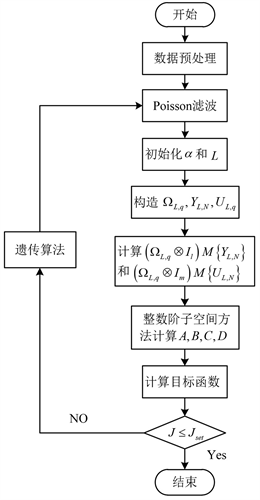Figure 3. Flow chart of fractional subspace identification algorithm

4. 仿真及结果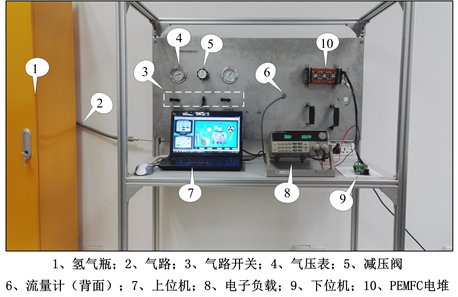Figure 4. Air-cooled self-humidifying PEMFC experimental platform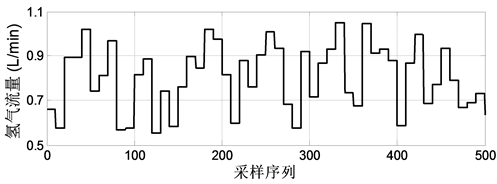Figure 5. Chart of hydrogen flow rate change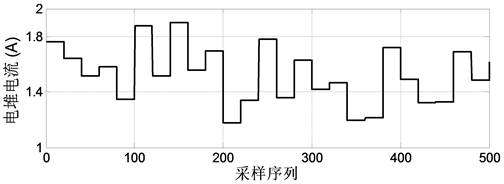Figure 6. Current change curve of reactor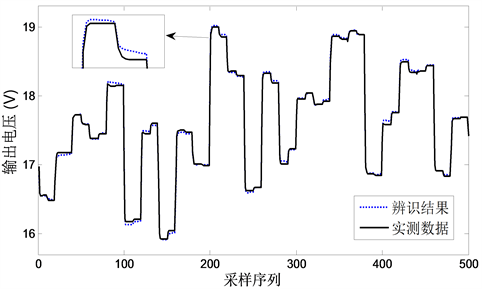Figure 7. Contrast diagram of output voltage results for fractional subspace identificationFigure 8. Contrast diagram of output power results of fractional subspace identification

$\alpha =0.947$

$A=\left[\begin{array}{ccc}0.9697& 0.2059& 0.0119\\ 0.0431& 0.5691& 0.5883\\ -0.0912& 0.2284& 0.6630\end{array}\right]$ , $B=\left[\begin{array}{cc}-0.0496& -0.0332\\ -1.0696& 0.1410\\ 0.6745& -0.0822\end{array}\right]$

$C=\left[\begin{array}{ccc}-0.1522& 0.8536& -0.9611\\ -1.4070& -1.5883& -0.5296\end{array}\right]$ , $D=\left[\begin{array}{cc}-2.1070& 0.0013\\ 14.8263& 0.0021\end{array}\right]$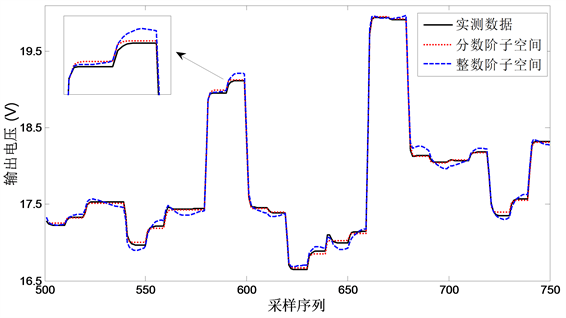Figure 9. Contrast diagrams between predicted output voltage and measured data of different identification algorithms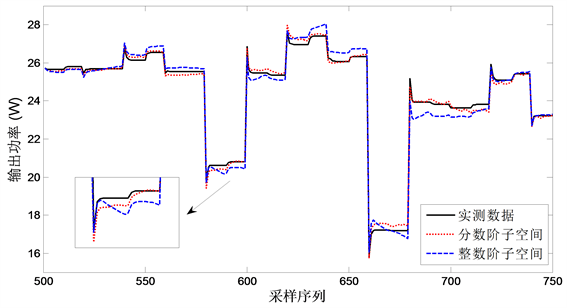Figure 10. Contrast diagrams between predicted output power and measured data of different identification algorithms

5. 总结

 Amphlett, J.C., Mann, R.F. and Peppley, B.A. (1996) A Model Predicting Transient Responses of Proton Exchange Membrane Fuel Cells. Journal of Power Sources, 61, 183-188.

 朱柳, 朱新坚, 沈海峰. 水冷型PEMFC的热管理研究[J]. 电源技术, 2012, 36(11): 1620-1622.

 Zhang, L., Mu, P. and Quan, S. (2008) Model Predictive Control of Water Management in PEMFC. Journal of Power Sources, 180, 322-329.

 杜新, 胡扬, 王金龙. 考虑水膜结构的PEMFC团聚体二相流模型[J]. 长春理工大学学报(自然科学版), 2016, 39(3): 70-72.

 Bao, C. and Bessler, W.G. (2015) Two-Dimensional Modeling of a Polymer Electrolyte Membrane Fuel Cell with Long Flow Channel. Part I. Model Development. Journal of Power Sources, 275, 922-934.

 Bao, C. and Bessler, W.G. (2015) Two-Dimensional Modeling of a Polymer Electrolyte Membrane Fuel Cell with Long Flow Channel. Part II. Physics-Based Electrochemical Impedance Analysis. Journal of Power Sources, 278, 675-682.
https://doi.org/10.1016/j.jpowsour.2014.12.045

 鲁聪达, 毛潘泽, 文东辉. PEMFC树状分形流场传递过程的数值分析[J]. 电源技术, 2016, 40(3): 565-568.

 张宁, 张小娟. 阴极扩散层孔隙率不同分布对PEMFC性能的影响[J]. 电源技术, 2017, 41(9): 1296-1298.

 Bian, H., Leng, B. and Shan, L. (2015) Research on Electrical Characteristics of State Space Modeling and Parameter Identifying of PEMFC. Proceedings of the 34th Control Conference, Hangzhou, 28-30 July 2015, 24.

 Qi, Z., Xu, S. and Liang, S. (2015) Dynamic Thermal Modeling of PEMFC Based on Fractional Order Theory. Proceedings of the 27th Control & Decision Conference, Qingdao, 23-25 May 2015, 4069-4072.

 张明. 基于分数阶微积分的PEMFC建模与辨识[D]: [硕士学位论文]. 北京: 北京化工大学, 2012.

 Taleb, M.A., Béthoux, O. and Godoy, E. (2016) Identification of a PEMFC Fractional Order Model. International Journal of Hydrogen Energy, 42, 1499-1509.

 胡聪. PEMFC分数阶状态空间建模与自适应控制研究[D]: [硕士学位论文]. 南京: 南京理工大学, 2017.

Top